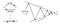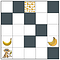# Reinforcement Learning Digest Part 2: Bellman Equations, Generalized Policy Iteration and Monte Carlo

In the last article, I have introduced Reinforcement learning Markov Decision Process (MDP) framework, discounted expected rewards and value and policy functions definitions. In this article, we will continue the definition of the MDP framework explaining Bellman and Bellman optimality equations. Additionally we will have describe our first reinforcement learning algrithm: Monte Carlo. So let us start…

# Bellman equations

In the last article, value and Q functions were defined as:

One important property of value and Q functions is that they can be expressed recursively. The value of state at time-step t can be expressed in terms of time-step t+1:

the last equation can rewritten as:V function Bellman equation

Similarly, Q-function can be expressed as:Q function Bellman equation

# Bellman Optimality equations

Now we have learnt about value and policy functions and bellman equations, we can start defining optimal value and policy functions. By optimal here we mean that they lead our agent to achieve its goal which is maximizing discounted cumulative returns.

Since value function is expressed as the expected discounted returns of states, this can lead us to a very logical definition of optimal policy with respect to value function:

Policy π is said to be better equal to policy π’ if and only if the

Please note that there can be more than one optimal policies. Optimal policy have associated value functions which can be expressed as:

As we have seen previously, Q-function for policy π expresses the expected cumulative returns for taking action a in state s and following the policy thereafter. It must then follow that the optimal value function can be defined as taking the maximum action on state s and following the optimal policy thereafter. Therefore, the optimal value function can be expressed as follows:

Then by following Q-function Bellman equation. the optimal value fucntion can be rewritten as:Bellman Optimality equation — value function

Similarity, Q- function can expressed in since q∗ gives expected return for taking an action a in state s and follow the optimal policy thereafter:

Then by substitution of v* by LHS of eq 1, we can express Q* as follows:Bellman Optimality equation — q function

From the last Bellman optimality equation, we can observe that the policy associated with optimal q function is indeed greedy as it selects the action with maximal value. But since the value function incorporates the expected cumulating future rewards, this simple greedy policy expressed by the Bellman equation is indeed also optimal in the long term. This is significant, as well see that a family of simple algorithms will be based on this greedy property of the Bellman optimality equation.

# Generalized Policy IterationGeneralized Policy Iteration (GPI)

Almost all reinforcement learning algorithms follow policy iteration in their way to oprimality. In this discussion let us assume that reinformcent learning algorithm is on-policy which is defined as using the policy being improved to generate experiences. Each iteration consists of two tasks: policy evaluation and policy improvement. During policy evaluation, the value function is aims at making the value function to be consistent with policy and during policy improvement the policy is improved by making it greedy with respect to the value function(but policy remains soft such π(a|s)>0 a ∈A, s ∈S to allow for exploration as we will see in next section). Thus policy iteration can be summarized as follows:

# Reinforcement Learning Algorithms

## Monte Carlo (on policy)

Monte Carlo on policy reinforcement algorithm is one of the simplest RL algrithims. The algorithm is called on policy because the same policy being improved is also used to interact withe environment during policy evaluation process.

Monte Carlo GPI: The main idea of Monte Carlo algorithm is to drive value function for every state-action pair to the average of expected returns observed in episodes(policy evaluation) and then drive policy to be greedy by selecting actions with maximum values for every state.

## Exploration vs Exploitationexploration vs exploitation

The above description makes realize a dilemma RL algorithms face: The need to optimize policy by making them greedy with respect to value function; but the same policy is also used to interact with environment and get experiences. If the policy used is made deterministically greedy during policy improvement, the algorithm will fail to visit several state-action pairs impeding its ability to discover optimal pairs. For instance, the monkey in grid can make go one step up and eat a single bannana and thus receive +1 reward. If we make the policy deterministically greedy, the monkey will tend to be greedy and always exploit the information he learnt and keep going one step up every episode to collect a single banana. This will cause the monkey agent to miss states with much rewarding returns. So it is important for the policy being improved in on policy algorithms to have non zero probabilty for all state-action pairs to allow the agent to continue exploring:

This condition can be achieved by using ϵ-Greedy, where ϵ is the exploration ratio. ϵ-Greedy will select greedy action leading to maximal expected returns most of the time, but with probability ϵ select random action to enable exploration. ϵ-Greedy policy applies to almost all on policy algorithms and not just Mote Carlo.

Now, we are ready to see Monte Carlo implementation.

In next article, we will explore different reinforcement algorithms that do not require to wait for a complete episode to finish to start learning.

Technical lead of IBM Cognos recommenders system